Welcome to WebmasterWorld Guest from 18.208.211.150

Forum Moderators: ocean10000

Message Too Old, No Replies

# Overflow error while doing simple subtraction in a VB Script

## VB Script - Overflow while subtract

#### jjforVBS

4:37 am on Aug 21, 2009 (gmt 0)

#### New User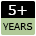joined:Aug 21, 2009
posts:3

Hi all,
I have got a very strange problem while running a VB script.
My VB script works without any error in normal case. However, if I open a particular window (graphic window) of my application, minimize and then run this VB script, I will get an error number 6, that is the overflow error.

My code is:
If (Err.Number <> 0) Then
Err.Clear

Dim x_min, x_max, xdiff

x_min = myfunction()� value is -600 in both cases
x_max = myfunction()� value is +600 in both cases

MsgBox("x_max = "& x_max & " and x_min = " & x_min) �prints same in both the cases ie 600 & -600

xdiff = x_max - x_min

MsgBox( xdiff ) � prints 1200 in normal case, no value in the abnormal case
MsgBox("Error number after xdiff = " & Err.Number) � prints 0 in normal case, 6 in the abnormal case

x_min and x_max is same for both the cases.

Can anybody help with any suggestions?

JJ

#### Ocean10000

7:38 pm on Aug 21, 2009 (gmt 0)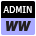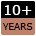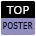joined:Jan 14, 2004
posts:864

You are hitting a divide by Zero error. I am guessing you are dividing by some input which in your abnormal case results in a value being divided by zero.

#### jjforVBS

4:42 am on Aug 24, 2009 (gmt 0)

#### New Userjoined:Aug 21, 2009
posts:3

Hi Ocean,

Thanks v much for the feedback.

The error happens only when I call xdiff = x_max - x_min (there is no division). Until there, Err.Number value is zero. After this call Err.Number becomes 6.

This happens only when my application's particular window (graphic) opened and minimized, otherwise it works fine.

Regards,
JJ

#### marcel

4:08 pm on Aug 24, 2009 (gmt 0)

#### Senior Member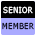joined:Feb 1, 2005
posts:732

what does myfunction() actually do?

* Edit - Nevermind, I see that you are sending the results to a messagebox... :)

#### jjforVBS

5:36 am on Aug 25, 2009 (gmt 0)

#### New Userjoined:Aug 21, 2009
posts:3

myfunction fetch the details of BoundingBox (x_min, x_max, y_min,y_max,z_min,z_max), which is specific to graphic.

Here if I assign values to x_min and x_max in the problem scenario, it works fine. When I get values using myfunction and then subtract like xdiff = x_max - x_min, the error pops up.

The confusing thing is, it prints the value correctly in the problem scenario before subtract (ie 600 & -600).

Step that prints values as shown below:
MsgBox("x_max = "& x_max & " and x_min = " & x_min) �prints same in both the cases ie 600 & -600

#### Hire Expert Members